[Next] [Up] [Previous] [Index]

# The Boomerang Attack

Recently, a means of improving the flexibility of differential cryptanalysis was discovered by David A. Wagner. Called the boomerang attack, it allows the use of two unrelated characteristics for attacking two halves of a block cipher.This diagram shows how the attack might work if everything goes perfectly for a particular initial block. The numbered points in the diagram show the steps involved in the attack.

1. Start with a random block of plaintext. Based on the characteristic known for the first half of the cipher, if we XOR a certain vector with it, called d1 (equal to 00100000 in the diagram), the result after half-enciphering the two plaintext blocks, before and after the XOR, will differ by c1 (equal to 00110110 in the diagram), if what we wish to learn about the key happens to be true.
2. Since the characteristic applies only to the first half of the cipher, the results after the whole block cipher won't be related. Take those two results, and XOR each one with d2 (equal to 01001011 in the diagram), which is the vector corresponding to the characteristic for the second half of the cipher. In each case, XORing d2 with a ciphertext block is expected to change the result after deciphering halfway by c2 (equal to 00010000 in the diagram), again, if something is true of the key.
3. With two intermediate results that differ by c1, if each one has c2 XORed to it, the two results of the XOR will still differ by c1. Since this difference now relates to the first half characteristic, it can be seen in the final output, thus indicating the truth or otherwise of two hypotheses about the key.

This increases the potential effectiveness of differential cryptanalysis, because one can make use of characteristics that do not propagate through the complete cipher. Also, certain kinds of added complexities, such as a bit transpose in the middle of the cipher, do not serve as a barrier to this method, since two values differing by an XOR with some value merely differ by an XOR with some other value after a bit transpose.

However, it has its limitations. It only produces a result if both characteristics are present; it does not allow testing for each characteristic independently. Even so, it seems to double the number of rounds a cipher needs to be considered secure.

Since at one end of a sequence of rounds, the precise difference between blocks that is required for the characteristic must be input, it isn't possible directly to cascade this method to break a block cipher into four or more pieces.

Note that any single Feistel round has a large family of "characteristics" that is 100% probable, but which tells nothing about the key, since any pattern that involves leaving the half that is input to the F-function unchanged, but involves an XOR to the half that is XORed with the output of the F-function applies, so one of the things this method can do is allow the use of attacks against the first or last 15 rounds of DES against 16-round DES. Hence, if by some other trick a block cipher with 16 rounds could be broken into 16 pieces like this, one could test for an informative characteristic which applied to any single round.

### The Boomerang Amplifier Attack

A technique called the boomerang amplifier attack works like this: instead of considering the pairs of inputs, differing by the XOR required for the characteristic of the first few rounds, as completely independent, one could note that it would be quite likely that somehow, taking two such pairs at a time, one could obtain any desired XOR difference between two such pairs by the birthday paradox. This allows a boomerang attack to be mounted with only chosen plaintext, instead of adaptive chosen ciphertext as well.

I wondered if one could use the boomerang amplifier technique noted above to allow breaking a block cipher up into three pieces instead of two.

First, you start by enciphering a large number of chosen plaintext pairs, differing by the XOR amount required for the characteristic of the first piece. By the birthday paradox, there will be a good chance of some pair of two of those pairs, somewhere among that number, which differ by the right amount to engage the differential characteristic of the middle piece.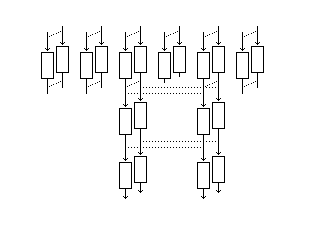I then take all the outputs of this process, and XOR them by the quantity required to engage, upon decipherment, the characteristic of the third piece.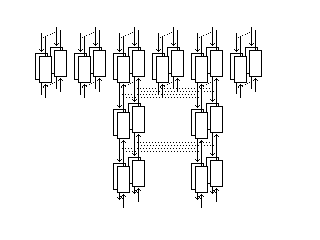Doing so ensures that the corresponding two pairs of blocks also has the XOR amount for the characteristic of the middle piece, this time in the reverse direction, as can be seen more clearly when we look at the following diagram of the upwards journey by itself.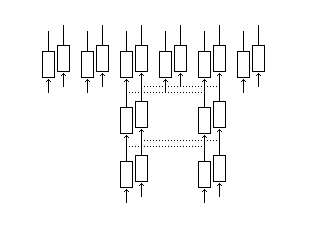Unfortunately, though, the thing about a differential characteristic is that it only refers to the XOR between two blocks, and not the values of the blocks.

If a characteristic implies that A xor B equals X xor Y, and equals the characteristic, then it is true that A xor X and B xor Y are equal, but the value to which both of them are equal could have any value. Hence, we have not preserved any structure that implies that we will have the correct differential for the first piece, during decipherment.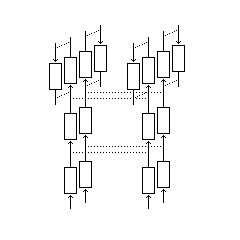Well, we can still apply the differential for the first piece, and then continue in the reverse order again.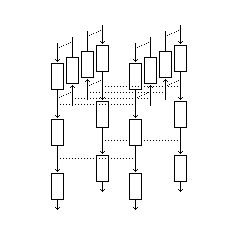But we run into the same problem; we have no characteristic preserved on output. So it appears that breaking a block cipher into three parts is hopeless. But then we notice that, by iterating in this fashion over our large number of input pairs, we can indefinitely preserve the characteristic in the middle.

This would only work if the characteristics involved had probability one, or very nearly one. Assuming that somehow this could be overcome, though, since one has produced a large number of pairs, in the same spot within our large number of pairs, that have the middle differential activated, if one of the elements of each of two pairs differs from the same element in another cycle by the right amount for the top differential, then the one connected with it by the middle differential will also match, not the other member of the same pair, and this is how the two pairs involved with the middle differential can finally be distinguished.

But because the birthday paradox just says that, to find two matching values for a block having N values, you only need a number of blocks proportional to the square root of N. Using the birthday paradox twice means that the complexity of this attack is proportional to the square root of N times itself, in other words, to N, and so this attack, even if it were possible, has a complexity equivalent to that of the codebook attack: just use as chosen plaintext every possible input block, and record the result.

[Next] [Up] [Previous] [Index]